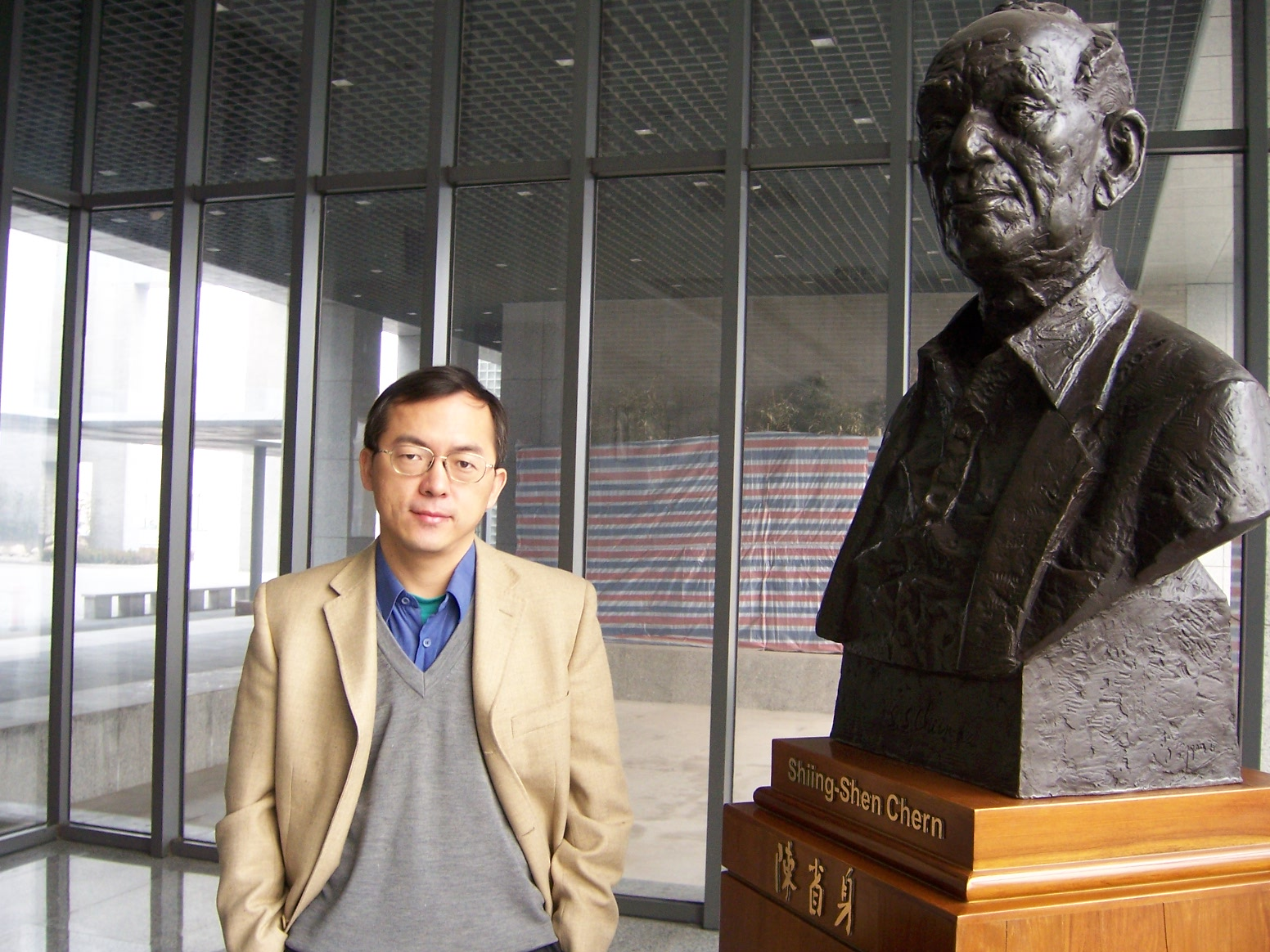﻿ 张伟平-陈省身数学研究所教师资源信息

Weiping Zhang

Researcher in Mathematics

Chern Institute of Mathematics
Nankai University
Tianjin 300071
People's Republic of China

Tel. +86-22-23500760

Fax +86-22-23501532

******************************

Member of the Chinese Academy of Sciences (Elected 2007)
（中国科学院院士）

Member of TWAS (Elected 2001)
（第三世界科学院（现改为“发展中国家科学院”）院士）

Email: weiping@nankai.edu.cnEducation:

1981.9--1985.7: Dept. of Math., Fudan Univ., Shanghai;

1985.9--1988.7: Inst. of Math., Academia Sinica, Beijing. Master Degree with advisor Professor Yu Yanlin;

1988.9--1990.6: As a Ph. D. student in Nankai Inst. of Math., Tianjin, with advisor Professor Shiing-shen Chern;

1990.7--1993.6: Dept. de Math., Univ. Paris-Sud, France. Ph. D. Degree with advisor Professor Jean-Michel Bismut;

2008.10.10: Dept. de Math., Univ. Paris-Sud, France. Habilitation a diriger des recherches.

Work Experience:

1993.9--present: Chern  Institute of Mathematics, Nankai University, Tianjin. As Professor since 1995.6

2004.1--2007.12: Director of the Nankai Institute of Mathematics (name changed to the Chern Institute of Mathematics on December 3rd of 2005)

=========================================

Visits:

1992.7--1993.6: IHES, France;

1994.1--1994.6: MSRI, Berkeley, USA;

1995.9--1996.8: Courant Inst. of Math. Sci., New York, USA;

1998.12--1999.1: IHES, France;

2000.6.10--2000.7.9: Humboldt-Univ. zu Berlin, Germany;

2001.1--2001.5: Dept. of Math., MIT, USA;

2005.2--2005.3: IHES, France;

2006.12.4--2006.12.16: CMS of ANU, Australia;

2007.3: Lab. Emile Picard, Univ. Paul Sabatier, France;

2008.4.15--2008.5.15: East China Normal University,
Shanghai, China;

2008.5.20--2008.7.20: Ecole Polytechnique, France;

2008.11--2008.12: Fudan University, Shanghai, China;

2009.12.6--2009.12.12: University of Tokyo, Japan;

2011.2.10--2011.3.10: Univ. Paris 7, France;

2014.5.22--2014.5.29: Univ. of Porto Rico, USA;

2015.3.1--2015.3.10: Univ. Texas A&M, USA;

2015.6.22--2015.7.2: Univ. Paris 7, France.

Research Interest:

Geometry and analysis on manifolds.

Bibliography:

1. Local index theorem of Atiyah-Singer for families of Dirac operators. Lect. Notes in Math. vol. 1369, pp. 351-366. Springer-Verlag, 1989.

2. A remark on a residue formula of Bott. Acta Math. Sinica (N.S.) 6 (1990), 306-314.

3. (with J. D. Lafferty and Y. Yu) Clifford asymptotics and the local Lefshetz index. Lect. Notes in Math. vol. 1411, pp. 137-142. Springer-Verlag, 1990.

4. A note on equivariant eta invariant. Proc. Amer. Math. Soc. 108 (1990), 1121-1129.

5. (with J.-M. Bismut) Metrique de Reidemeister et m'etrique de Ray-Singer sur le determinant de la cohomologie d'un fibre plat: une extension d'un resultat de Cheeger-Mueller. C. R. Acad. Sci. Paris, Serie I, 313 (1991), 775-782.

6. (with J. D. Lafferty and Y. Yu) A direct geometric proof of the Lefschetz fixed point formula. Trans. Amer. Math. Soc. 329 (1992), 571-583.

7. Eta invariants and Rokhlin congruences.  C. R. Acad. Sci. Paris, Serie I, 315 (1992), 305-308.

8. (with J.-M. Bismut) An extension of a theorem by Cheeger and Mueller. Asterisque, tom. 205, Soc. Math. France, 1992.

9. Elliptic genera and Rokhlin congruences. Preprint, IHES/M/92/76.

10. (with J.-M. Bismut) Real embeddings and eta invariant. Math. Ann. 295 (1993), 661-684.

11. A proof of the mod 2 index theorem of Atiyah and Singer. C. R. Acad. Sci. Paris, Serie I, 316 (1993), 277-280.

12. Spin^c-manifolds and Rokhlin congruences. C. R. Acad. Sci. Paris, Serie I}, 317 (1993), 689-692.

13. A remark on the regularity of the eta function of Dirac operators. Acta Math. Sinica (N. S.) 9 (1993), 240-245.

14. Circle bundles, adiabatic limits of eta invariants and Rokhlin congruences. Ann. Inst. Fourier 44 (1994), 249-270.

15. (with J.-M. Bismut) Milnor and Ray-Singer metrics on the equivariant determinant of a flat vector bundle. Geom.  Funct. Anal. 4 (1994), 136-212.

16. (with K. Liu) Elliptic genera and eta-invariant. Inter. Math. Res. Notices  No. 8 (1994), 319-327.

17. (with X. Dai) The Atiyah-Patodi-Singer index theorem for manifolds with boundary: a proof using embeddings. C. R. Acad. Sci. Paris, Serie I, 319 (1994), 1293-1297.

18. A mod 2 index theorem for pin^- manifolds. Preprint, MSRI 053-94.

19. (with X. Dai) Circle bundles and the Kreck-Stolz invariant. Trans. Amer. Math. Soc. 347 (1995), 3587-3593.

20. On the mod k index theorem of Freed and Melrose. J. Diff. Geom. 43 (1996), 198-206.

21. (with X. Dai) Higher spectral flow. Math. Res. Lett. 3 (1996), 93-102.

22. The existence of Riemannian metrics with positive scalar curvature over complex hypersurface. Acta Math. Sinica (N. S.) 39 (1996), 460-462. (in Chinese)

23. Sub-signature operator and its local index theorem. Chinese Sci. Bull. 41 (1996), 294-295. (in Chinese)

24. (with X. Dai) Splitting of the family index. Commun. Math. Phys. 182 (1996), 303-318.

25. (with Y. Tian) Symplectic reduction and quantization. C. R. Acad. Sci. Paris, Serie I, 324 (1997), 433-438.

26. (with S. Wu) Equivariant holomorphic Morse inequalities III: non-isolated fixed points. Geom.  Funct. Anal. 8 (1998), 149-178.

27. (with Y. Tian) An analytic proof of the geometric quantization conjecture of Guillemin-Sternberg. Invent. Math. 132 (1998), 229-259.

28. (with X. Dai) Higher spectral flow. J. Funct. Anal. 157 (1998),  432-469.

29. (with Y. Tian) Holomorphic Morse inequalities in singular reduction. Math. Res. Lett. 5 (1998), 345-352.

30. (with Y. Tian) Symplectic reduction and a weighted multiplicity formula for  twisted Spin^c-Dirac operators. Asian J. Math. 2 (1998), 591-608.

31. Analytic and topological invariants associated to nowhere zero vector fields. Pacific J. Math. 187 (1999), 379-398.

32. (with Y. Tian) Quantization formula for symplectic manifolds with boundary. Geom. Funct. Anal. 9 (1999), 596-640.

33. Holomorphic quantization formula in singular reduction. Commun. Contemp. Math. 1 (1999), 281-293.

34. Symplectic reduction and family quantization. Inter. Math. Res. Notices (1999), No. 19, 1043-1056.

35. A mod 2 index theorem for the twisted Signature operator. Science in China (Series A) 42 (1999), 1279-1285.

36. A counting formula for the Kervaire semi-characteristic. Topology 39 (2000), 643-655.

37. (with K. Liu and  X. Ma) Rigidity and vanishing theorems in K-theory. C. R. Acad. Sci. Paris, Serie I, 330  (2000), 301--306.

38. $\eta$-invariants and the Poincare-Hopf index formula. Geometry and Topology of Submanifolds X, pp. 336-345. World Scientific, Singapore, 2000.

39. (with X. Dai) Real embeddings and the Atiyah-Patodi-Singer index theorem for Dirac operators. Asian J. Math. 4 (2000), 775-794.

40. (with K. Liu and X. Ma) Spin$^c$ manifolds and rigidity  theorems in $K$-theory. Asian J. Math. 4 (2000), 933-960.

41. (with K. Liu) Adiabatic limits and foliations. Contemp. Math. 279 (2001), 195-208.

42. (with K. Liu and X. Ma) On elliptic genera and foliations. Math. Res. Lett. 8 (2001), 361-376.

43. (with H. Feng and W. Hu) A family quantization formula for symplectic manifolds with boundary. Science in China (Series A), 44 (2001), 599-609.

44. Lectures on Chern-Weil Theory and Witten Deformations. World Scientific, Singapore, 2001.

45 Heat kernels and the index theorems on even and odd dimensional manifolds. Proc. ICM2002, Vol. 2, pp. 361-369.

46. (with Z. Tang) A generalization of the Atiyah-Dupont vector fields theory. Commun. Contemp. Math. 4 (2002), 777-796.

47. (with K. Liu and X. Ma) Rigidity and vanishing theorems in $K$-theory. Commun. Anal. Geom. 11 (2003), 121-180.

48. (with F. Han) Spin^c-manifolds and elliptic genera. C. R. Acad. Sci. Paris, Ser. I, 336 (2003), 1011-1014.

49. Circle actions and ${\bf Z}/k$-manifolds. C. R. Acad. Sci. Paris, Ser. I, 337 (2003), 57-60.

50. Sub-signature operators, $\eta$-invariants and a Riemann-Roch theorem for flat vector bundles. Chin. Ann. Math. 25B (2004), 7-36.

51. (with F. Han) Modular invariance, characteristic numbers and $\eta$ invariants. J. Diff. Geom. 67 (2004), 257-288.

52. $\eta$-invariant and Chern-Simons current. Chin. Ann. Math. 26B (2005), 45-56.

53. An extended Cheeger-Mueller theorem for covering spaces. Topology 44 (2005), 1093-1131.

54. (with X. Ma) Bergman kernels and symplectic reduction. C. R. Acad. Sci. Paris, Serie I, 341 (2005), 297-302.

55. (with X. Ma) $\eta$-invariant and flat vector bundles. Chin. Ann. Math. 27B (2006), 67-72.

56 (with W. Li) An $L^2$-Alexander invariant for knots. Commun. Contemp. Math. 8 (2006), 167-187.

57. (with X. Ma) An anomaly formula for $L^2$-analytic torsions on manifolds with boundary. in  Analysis, Geometry and Topology of Elliptic Operators: Papers in Honor of Krzysztof P Wojciechowski. Eds. B. Booss-Bavnbek, S. Klimek, M. Lesch and W. Zhang,  World Scientific, 2006, pp. 247-274.

58. (Eds. with B. Booss-Bavnbek, S. Klimek and M. Lesch) Analysis, Geometry and Topology of Elliptic Operators: Papers in Honor of Krzysztof P Wojciechowski. World Scientific, 2006.

59. (with X. Dai) An index theorem for Toeplitz operators on odd dimensional manifolds with boundary. J. Funct. Anal. 238 (2006), 1-26.

60. (With W. Li) An $L^2$ Alexander-Conway invariant for knots and the volume conjecture. in Differential Geometry and Physics. Eds. M.-L. Ge and Weiping Zhang, Nankai Tracts in Mathematics Vol. 10, World Scientific, 2006, pp. 303-312.

61. (With X. Ma) Toeplitz quantization and symplectic reduction. in Differential Geometry and Physics. Eds. M.-L. Ge and Weiping Zhang, Nankai Tracts in Mathematics Vol. 10, World Scientific, 2006, pp. 343-349.

62. (Eds. with M.-L. Ge) Differential Geometry and Physics. Nankai Tracts in Mathematics Vol. 10, World Scientific, 2006.

63. (With X. Ma) Eta-invariant and flat vector bundles II. in Inspired by S. S. Chern. Ed. P. A. Griffiths, Nankai Tracts in Mathematics Vol. 11. World Scientific, 2006, pp. 335-350.

64. (with X. Ma) Superconnection and family Bergman kernels. C. R. Acad. Sci. Paris, Serie I, 344 (2007), 41-44.

65. (with X. Ma) Eta-invariants, torsion forms and flat vector bundles.  Math. Ann. 340 (2008), 569-624.

66. (with X. Ma) Bergman kernels and symplectic reduction. Asterisque, Tom. 318, Soc. Math. France, pp. 1-154,  2008.

67. (with Guangxiang Su) A Cheeger-Mueller theorem for symmetric bilinear torsions. Chin.  Ann. Math. 29B (2008), 385-424.

68. (with W. Li) Twisted $L^2$-Alexander-Conway invariants for knots. in Topology and Physics. Eds. Kevin Lin, Zhenghan Wang and Weiping Zhang, Nankai Tracts in Mathematics Vol. 12, World Scientific, 2008, pp. 236-259.

69. (Ed. with Kevin Lin and Zhenghan Wang) Topology and Physics. Nankai Tracts in Mathematics Vol. 12, World Scientific, 2008.

70. (with Huitao Feng and Guangbo Xu) Real embeddings, eta invariant and Chern-Simons current. Pure and Applied Mathematics Quarterly 5 (2009), 1113-1137.

71. (with X. Ma) Geometric quantization for proper moment maps. C. R. Math. Acad. Sci. Paris, Ser. I 347 (2009), 389-394.

72.  Cobordism and Rokhlin congruences. Acta Math. Scientia 29B (2009), 609-612.

73. (Ed. with X. Dai, R. Leandre and X. Ma) From Probability to Geometry (I), Volume in Hornor of the 60th Birthday of Jean-Michel Bismut. Asterisque Tom 327, 2009.

74. (Ed. with X. Dai, R. Leandre and X. Ma) From Probability to Geometry (II), Volume in Hornor of the 60th Birthday of Jean-Michel Bismut. Asterisque Tom 328, 2009.

75. (with Q. Chen and F. Han) Witten genus and vanishing results on complete intersections. C. R. Math. Acad. Sci. Paris, Ser. I 348 (2010), 295-298.

76. (with V. Mathai) Geometric quantization for proper actions (with an Appendix by U. Bunke).
Adv. in Math. 225 (2010), 1224-1247.

77. (with X. Dai) Adiabatic limit, Bismut-Freed connection, and the real analytic torsion form. J. reine angew. Math. 647 (2010), 87-113.

78. (with Q. Chen and F. Han) Generalized Witten genus and vanishing theorems.   J. Diff. Geom. 88 (2011), 1-40.

79. (with H. Feng and W. Li) A Poincaré-Hopf type formula for Chern character numbers. Math. Z. 269 (2011), 401-410.

80. (with J.-M.Bismut and X. Ma) Opérateurs de Toeplitz et torsion analytique asymptotique. C. R. Acad. Sci. Paris, Serie I. 349 (2011), 977-981.

81. (with F. Han and K. Liu) Modular forms and generalized anomaly cancellation formulas.    J. Geom. Phys. 62 (2012), 1038-1053.

82. (with X. Ma) Transversal index and L^2-index for manifolds with boundary. in
Metric and Differential Geometry, The Jeff Cheeger Anniversary Volume, pp. 299-315. Eds. Xianzhe Dai and Xiaochun Rong. Progress in Mathematics, vol. 297. Birkhäuser Boston, Inc., Boston, MA. 2012.

83. (with X. Tang and Y. Yao) Hopf cyclic cohomology and Hodge theory for proper actions.
Journal of Noncommutative Geometry 7 (2013), 885-905.

84.  (with Z. Tang) η-invariant and a problem of Bérard-Bergery on the existence of closed geodesics.   Advances in Mathematics  254 (2014), 41-48.

85. (with X. Ma) Geometric quantization for proper moment maps: the Vergne conjecture.   Acta Mathematica 212 (2014), 11-57.

86. (with F. Han and K. Liu) Anomaly cancellation and modularity.
in  Frontiers in Differential Geometry, Partial Differential Equations and Mathematical Physics (In Memory of Gu Chaohao).
Eds. Molin Ge, Jiaxing Hong, Tatsien Li and Weiping Zhang. World Scientific, 2014. pp. 87-104.

87. The mathematical work of V. K. Patodi.  Commun. Math. Stat. (2014), 253–277.

88. (with X. Dai) Eta invariant and holonomy: The even dimensional case. Advances in Mathematics 279 (2015), 201-306.

89. (with F. Han) η-Invariant and modular forms. The Quarterly Journal of Mathematics 66 (2015), 133–142.

90.  (with J.-M. Bismut and X. Ma) Asymptotic torsion and Toeplitz operators. Journal of the Institute of Mathematics of Jussieu 16 (2017), 223-349.

91.  Positive scalar curvature on foliations.  Annals of Mathematics 185 (2017), 1035-1068.

92. (with F. Han and K. Liu) Anomaly cancellation and modularity, II: the E8 × E8 case.  Science China Mathematics 60 (2017), 985-994.

93. A mod 2 index theorem for pin^- manifolds.  Science China Mathematics 60 (2017), 1615–1632.

94. A note on the Lichnerowicz vanishing theorem for proper actions. J. Noncommut. Geom. 11 (2017), 823–826.

95. (with J. Yu) Positive scalar curvature and the Euler class.  Journal of Geometry and Physics 126 (2018), 193-203.

96. (with G. Su) Positive scalar curvature and connected sums. Preprint, arXiv: 1705.00553v2. in Surveys in Geometric Analysis 2017. pp. 144-150.  Ed. G. Tian, Q. Han and Z. Zhang, Science Press, Beijing 2018.

97. Positive scalar curvature on foliations: the enlargeability. Progress in Math. 333 (2020), 537-544.

98. Nonnegative scalar curvature and area decreasing maps. SIGMA 16 (2020), 033, 7pages.

99. A note on the Kervaire semi-characteristic. Intern. J. Math. Vol. 32, No. 12 (2021) 2140004 (4 pages).

100. (with H. Feng) Flat vector bundles and open covers. Differential Equations on Manifolds and Mathematical Physics. V. M. Manuilov et al (eds), Trends in Mathematics 2021, pp. 142-155.

101. (with F. Han, R. Huang and K. Liu) Cubic forms, anomaly cancellation and modularity. Adv. in Math. 394 (2022), 108023 (46 pages).

102. (with H. Feng) Geometry and Analysis on Manifolds. (in Chinese) Higher Education Press, 2022. 《流形上的几何与分析》（张伟平、冯惠涛），高等教育出版社 2022.

103. (with G. Su and X. Wang) Nonnegative scalar curvature and area decreasing maps on complete foliated manifolds.  J. reine angew. Math. 790 (2022), 85-113.

104. (with X. Wang) A note on the generalized Geroch conjecture for complete spin manifolds. Preprint, arXiv2204.09184. To appear in Chinese Annals of Math.

105. (with F. Han and K. Liu) $E_8$ Bundles and Rigidity. Preprint, arXiv:1307.6137.

106. Dirac operators on foliations: a Lichnerowicz inequality. Preprint, arXiv:1204.2224v5.

107. (with G. Su) Positive scalar curvature on foliations: the noncompact case. Preprint, arXiv:1905.12919.

More......

More......